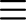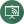LiveBoard• Use Cases
• Resources
• Pricing
• Blog

#### How To Teach Multiplication Tables To Kids in 7 Easy StepsLiveBoard Staff
28 June 2022
Math TutoringLiveBoard Staff

Teaching multiplication tables to kids can be a challenging task for many educators. However, it is essential to remember that with a bit of patience and creativity, it’s possible to make learning tables fun and engaging for kids of all ages.

Here are seven easy steps on how to teach times tables to kids in a way that is both effective and enjoyable:

## 1. Relate Multiplication to Addition

Before diving into the times table, your pupil must understand multiplication. Help them see that multiplication is simply repeated addition. For example, when we multiply 4 x 3, we are really just adding 4 + 4 + 4 (3 times).

Kids are better visual learners, so you might want to show them examples with familiar objects that might help them understand the concept more easily. Focusing solely on memorization would not be the best way to teach the multiplication table. You want children to fully grasp it, practice it, and only then memorize it.

For example, you can show them an example with cherries. If you’re using LiveBoard, you can insert images, duplicate them, and write and highlight text to showcase a basic multiplication as repeated addition, as in the example below:

The first numbers in the multiplication table are 0 and 1. The multiples of 0 are simply 0. Use real-world allegories to showcase this. For example, in a classroom with 15 tables with no books on them, there are 0 books on the tables.

Your pupils should have no problem understanding the multiples of 1. The multiples of 1 are just the numbers themselves. So, the multiples of 1 are 1, 2, 3, 4, 5, and so on. You can use a simple count to help kids understand this concept.

## 3. Continue With The “Easy” Numbers

By now your pupils have probably understood that multiplication means repeated addition. Before starting with other numbers, ensure they thoroughly understand how the multiplication works and have grasped multiplication by 0 and 1.

Now, it’s time to cover the multiplication table itself. Start with the small numbers like 2, 3, and 4. These are easy to multiply, and your pupils shouldn’t have any problem understanding them.

## 4. Explain The Commutative Property

One of the properties of multiplication is that it’s commutative. This means that the order of numbers doesn’t matter when multiplying. For example, 3 x 4 is the same as 4 x 3. This is also true of numbers further away from each other, like 2 x 9 equals 9 x 2.

The concept can be confusing for kids, so explain it to them clearly and give them plenty of examples. Additionally, have them write such examples themselves. It’s the easiest way to help them understand this concept better in the long term.

While teaching a larger classroom with LiveBoard, you can have each pupil write their examples on the digital board itself, as LiveBoard comes with an infinite canvas. Thus, everyone can work on the task simultaneously, and you can track their progress. Check the “drawing access for participants upon entry” box before sharing the board.

If you give your pupils simple tips on memorizing more complex multiplications in the table, they will have a much better time doing so. For example, any number multiplied by five either ends in a 5 or 0. To demonstrate, write down 1 x 5 = 5, 2 x 5 = 10, 3 x 5 = 15, 4 x 5 = 20, and so on.

Similarly, when you multiply a number by 9, the result is always 10 times the number minus the number. Demonstrate this too by writing down a few examples, like 1 x 9 = 10 – 1, 7 x 9 = 70 – 7. Also, explain to them how it works so they don’t get confused.

Take advantage of LiveBoard’s feature that allows you to add PDF files to your boards. You can import a 10×10 multiplication chart and explain it to the class. Click on the PDF icon on the toolbar and select the pages you want to import, whether they have none of the answers, or some, or even all of them.

## 6. Let Your Pupils Practice on a Multiplication Chart

One of the best ways to help children memorize multiplication tables would be to provide them with a half-filled or a blank multiplication chart, explain how it works, and allow them to practice.

The key here is to let the pupils practice as much as possible. The more times a child is exposed to something, the better they remember it. So, make sure your pupils practice as much as possible at home and in school.

With the PDF page imported in LiveBoard, pupils can fill their answers in the necessary spaces using either the text or drawing tool.

Now that your pupils have grasped the concept of multiplication and understand how it works, it’s time to help them memorize multiplication tables.

Your students may have already begun to memorize the multiplication table themselves, perhaps without realizing it. But the main point here is to explain why they need to do so and how they can apply it to real-world situations.

• Encourage your students to use a multiplication chart, practice as much as possible, and try different methods of memorization, such as saying the table out loud or writing it down. When they are ready, test them on small table sections at a time. As they progress, increase the size of the sections they are tested on.
• Introduce new multiplications gradually, instead of the entire table at once. Try to relate the new numbers with those that your students already know. For example, when introducing the 7-times table, point out they have already learned how to multiply previous numbers with 7 (1×7, 3×7, 6×7, etc.).
• Give pupils time to practice the new facts before moving on to the next stage. Repetition is key when memorizing multiplication tables.
• Approach memorizing tables in a strategic order. Start with the 0s and 1s, then from 2 to 3, from 4 to 7, and from 8 to 10. You can also start with the doubles (2×2, 3×3, 4×4, etc.), then move on to the near-doubles (2×3, 3×4, 4×5, etc.), and so on. However, make sure you’re consistent, methodical and patient to get the best results.

## Final Thoughts

We hope that you have found this guide on how to teach multiplication tables to kids useful. If you follow these seven easy steps, your pupils should be able to learn their multiplication tables in no time at all. Remember, practice makes perfect, so make sure your child spends plenty of time practicing what they have learned.